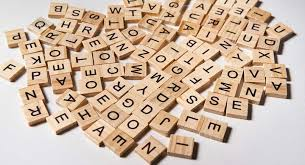# Can you decipher the code to open the door?

Have you tried puzzles where you need to decipher the code to open the door or some vault.

It is always fun to solve puzzles where you have to crack the code to unlock the lock. These type of puzzles need to some really sharp brain. Here is another such puzzle. Let’s see if you can solve it.

### Can you decipher the code to open the door?

There are 3 hints on the door in the form of shapes. You need to figure out number using these shapes, which can be used to open this door.

First shape is triangle with a straight line in middle, second shape is letter M and third shape is heart shape with horizontal straight line at bottom.

##### Hint: This number code is 6 digit number

Once you have tried and have a number to unlock the door then, you can scroll down to find the answer. Let’s see if you figured out the correct code.

If you are done solving this puzzle then, you can also try finding some Butterflies in this picture where 95% People Fail To Find All 3 Hidden Butterflies . I am sure it would be really interesting.

Code to unlock the door is 441122

Explanation:

First shape is triangle with a straight line in middle –> it is nothing but number 4 which is merged with its reverse number.

It is like 4 which is merged with 𐊀

similarly second shape is number 1 which is merged with ᛚ

Third shape is number 2 which is merged with its reverse